### Spoligotype Database of Mycobacterium tuberculosis: Biogeographic Distribution of Shared Types and Epidemiologic and Phylogenetic Perspectives

Christophe Sola*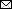, Ingrid Filliol*, Maria Cristina Gutierrez†, Igor Mokrousov*, Véronique Vincent†, and Nalin Rastogi*Author affiliations: *Institut Pasteur de Guadeloupe, Pointe à Pitre, Guadeloupe; †Centre National de Référence des Mycobactéries, Institut Pasteur, Paris, France

Main Article

#### Table 4

Analysis of distribution of shared types found in both USA and Europea

Europe
USA
World
No.
%b
No.
%

%
d/σd
Type (k1) (p1) (k2) (p2)  No. (p0) quotientc
1 21 1.8 326 25.5 476 14.4 15.3d
2 6 0.5 2 0.2 28 0.8 1.6
8 10 0.9 7 0.5 19 0.6 0.9
19 1 0.1 23 1.8 27 0.8 4.2d
20 8 0.7 2 0.2 20 0.6 2.1d
25 13 1.1 3 0.2 17 0.5 2.7d
26 22 1.9 5 0.4 28 0.8 3.6d
33 13 1.1 10 0.8 38 1.2 0.9
34 6 0.5 9 0.7 21 0.6 0.6
37 17 1.5 2 0.2 28 0.8 3.7d
44 12 1.1 1 0.1 15 0.5 3.3d
47 25 2.2 23 1.8 65 2.0 0.7
48 34 3.0 7 0.5 41 1.2 4.6d
50 56 4.9 32 2.5 155 4.7 3.1d
52 29 2.5 7 0.5 40 1.2 4.0d
53 79 6.9 46 3.6 218 6.6 3.6d
58 4 0.4 7 0.5 17 0.5 0.7
62 7 0.6 4 0.3 15 0.5 1.1
92 2 0.2 8 0.6 14 0.4 1.7
118 8 0.7 1 0.1 9 0.3 2.5d
119 2 0.2 110 8.6 115 3.5 9.6d
137 10 0.9 134 10.5 146 4.4 9.7d
138 5 1 1 0.1 6 0.2 1.8
139 19 1.7 19 1.5 38 1.2 0.3

aResults are given for 24 of 45 shared types that contained enough isolates to compare the results statistically.
bPercentages were calculated on the basis of 1,142 (n1), 1,276 (n2), and 3,319 individual spoligotypes reported, respectively, for Europe (p1), USA (p2), and the full database available for the world.
cThe quotient d/σd was calculated by using the equation d/σd=p1-p2/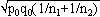, where d is the absolute value of the difference between p1 and p2, σd is the standard deviation of the repartition law of d which follows a normal distribution and can be calculated by the equation σd =, and where p0 is best estimated by the equation p0=k1+k2/n1+n2=n1p1+n2p2/ n1+n2. In this equation, individual sampling sizes are n1 and n1, the number of individuals within a given shared-type "x" are k1 and k2, and the representativeness for the two samples is p1=k1/n1 and p2=k2/n2.
dIf the absolute value of the quotient d /σd<2, the variations observed in the distribution of isolates for a given shared type were not statistically significant and could be due to a sampling bias. Inversely, if d /σd>2, then the differences observed in the distribution of isolates for a given shared type were statistically significant and not due to a potential sample bias.

Main Article

1For this purpose, the independent sampling sizes for Europe and the USA were taken as n1 and n2, the number of individuals within a given shared-type "x" was k1 and k2, and in this case, the representativeness of the two samples was p1=k1/n1 and P2=k2/n2, respectively. To assess if the divergence observed between p1 and p2 was due to sampling bias or the existence of two distinct populations, the percentage of individuals (p0) harboring shared-type "x" in the population studied was estimated by the equation p0= k1+k2/n1+n2=n1p1+n2p2/n1+n2. The distribution of the percentage of shared-type "x" in the sample sizes n1 and n2 follows a normal distribution with a mean p0 and a standard deviation of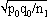and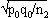respectively, and the difference d=p1-p2 follows a normal distribution of mean p0-p0=0 and of variance σd2p12p22 = p0q0/n1+p0q0/n2 or σd2=p0q0 (1/n1+1/n2). The two samples being independent, the two variances were additive; the standard deviation σd= was calculated, and the homogeneity of the samples tested was assessed using the quotient d/σd=p1-p2/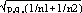. If the absolute value of the quotient d/σd<2, the two samples were considered to belong to a same population (CI 95%) and the variation observed in the distribution of isolates for given shared types could be due to a sampling bias. Inversely, if d/σd>2, then the differences observed in the distribution of isolates for given shared types were statistically significant and not due to potential sample bias.

Page created: April 26, 2012
Page updated: April 26, 2012
Page reviewed: April 26, 2012
The conclusions, findings, and opinions expressed by authors contributing to this journal do not necessarily reflect the official position of the U.S. Department of Health and Human Services, the Public Health Service, the Centers for Disease Control and Prevention, or the authors' affiliated institutions. Use of trade names is for identification only and does not imply endorsement by any of the groups named above.